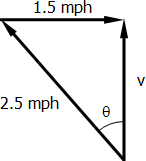# river

2 posts / 0 new
Lyneth Foman-egriver

A river flows 1.5 miles an hour.(a) In what direction must a man swim in order to go straight across if his rate of swimming in still water is 2.5 miles an hour?(b) How long will it take him to cross the river if it is 1/ mile wide?

Rate:
Jhun VertPart (a)
$\sin \theta = \dfrac{1.5}{2.5}$

$\theta = 36.87^\circ$   ←   against the flow of the river$v^2 + 1.5^2 = 2.5^2$

$v = 2 ~ \text{mph}$

$t = \dfrac{s}{v} = \dfrac{1}{2}$

$t = 0.5 ~ \text{hr} = 30 ~ \text{min}$

Rate: